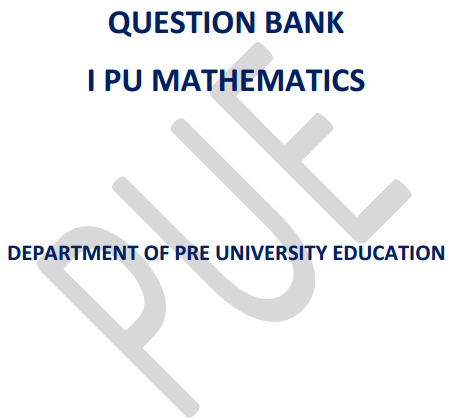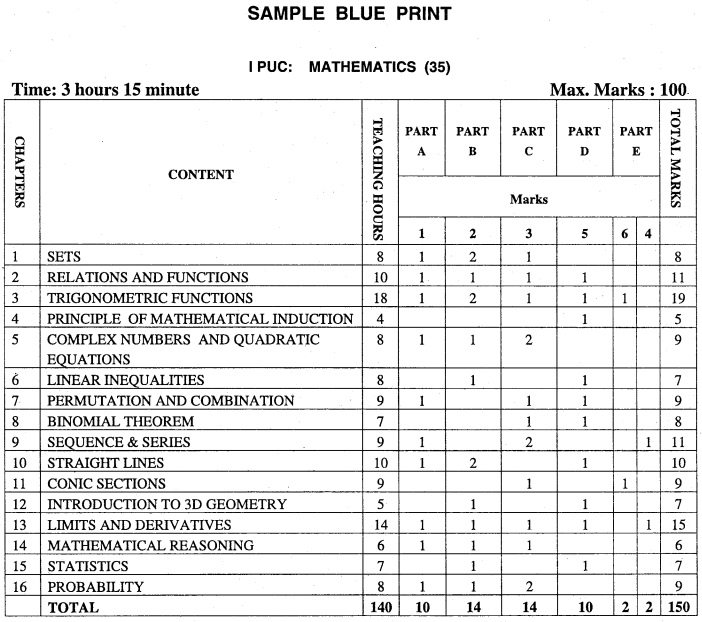# 1st PUC Maths Question Bank with Answers Karnataka

Chapterwise 1st PUC Maths textbook solutions karnataka Board are given here in PDF format for understanding the concepts involved in the syllabus. If you are looking for Chapter-wise KSEEB Solutions for 1st PUC Maths Question Bank with Answers Solutions, Notes, Guide Pdf are given in Chapter wise format then this is the right page for you. Students are advised to download chapter wise 1st PUC Question Bank with Answers Karnataka solutions pdf to take their preparation to the next level. Go through this page and get all chapters pdf links of KSEEB solutions for 1st PUC Maths textbook solutions pdf & learn efficiently to score high marks.

Students can also read 1st PUC Maths Model Question Papers with Answers hope will definitely help for your board exams.## Karnataka 1st PUC Maths Question Bank with Answers

By taking the help of the best resources like Karnataka State Board Solutions for 1st PUC Maths Textbook Questions and Answers you all can easily understand the concepts involved in the latest KTBS Solutions are given in syllabus and gain more subject knowledge. Study 1st PUC Maths Question Bank with Answers Karnataka solutions on a daily basis and be confident to answer all the questions asked in the final exam. Chapter-wise KSEEB solutions for 1st PUC Maths PDF links are available below to download.

### Karnataka 1st PUC Maths Syllabus and Marking Scheme

Karnataka 1st PUC Maths Blue Print of Model Question PaperInstructions:
Content area to select questions for PART D and PART E
(a) In PART D

1. Relations and functions: Problems on drawing graph of a function and writing its domain and range.
2. Trigonometric functions: Problems on Transformation formulae.
3. Principle of Mathematical Induction: Problems.
4. Permutation and Combination: Problems on combinations only.
5. Binomial theorem: Derivation/problems on Binomial theorem.
6. Straight lines: Derivations.
7. Introduction to 3D geometry: Derivations.
8. Limits and Derivatives: Derivation / problems.
9. Statistics: Problems on finding mean deviation about mean or median.
10. Linear inequalities: Problems on solution of system of linear inequalities in two variables.

(b) In PARTE

6 mark questions must be taken from the following content areas only.

1. Derivations on trigonometric functions.
2. Definitions and derivations on conic sections.

4 mark questions must be taken from the following content areas only.

1. Problems on algebra of derivatives.
2. Problems on summation of finite series.

Unit-I: Sets and Functions

Chapter 1 Sets:
Sets and their representations. Empty set. Finite and Infinite sets. Equal sets. Subsets. Subsets of a set of real numbers especially intervals (with notations). Power set. Universal set. Venn diagrams. Union and Intersection of sets. Difference of sets. Complement of a set. Properties of Complement Sets. Practical Problems based on sets. (8 Hours)

Chapter 2 Relations & Functions:
Ordered pairs, Cartesian product of sets. Number of elements in the cartesian product of two finite sets. Cartesian product of the sets of real (upto R x R). Definition of relation, pictorial diagrams, domain, co-domain and range of a relation. Function as a special kind of relation from one set to another. Pictorial representation of a function, domain, co-domain and range of a function. Real valued functions, domain and range of these functions: constant, identity, polynomial, rational, modulus, signum, exponential, logarithmic and greatest integer functions, with their graphs. Sum, difference, product and quotients of functions. (10 Hours)

Chapter 3 Trigonometric Functions
Positive and negative angles. Measuring angles in radians and in degrees and conversion of one into other. Definition of trigonometric functions with the help of unit circle. Truth of the sin2x+cos2x=1, for all x. Signs of trigonometric functions. Domain and range of trignometric functions and their graphs. Expressing sin (x±y) and cos (x±y) in terms of sin x, sin y, cos x & cos y and their simple application. Deducing identities like the following:

Identities related to sin 2x, cos 2x, tan 2x, sin 3x, cos 3x and tan 3x. General solution of trigonometric equations of the type sin y = sin a, cos y = cos a and tan y = tan a and problems. Proofs and simple applications of sine and cosine rule. (18 Hours)

Unit-II: Algebra

Chapter 4 Principle of Mathematical Induction:
Process of the proof by induction, motivating the application of the method by looking at natural numbers as the least inductive subset of real numbers. The principle of mathematical induction and simple applications. (4 Hours)

Chapter 5 Complex Numbers and Quadratic Equations:
Need for complex numbers, especially √1, to be motivated by inability to solve some of the quardratic equations. Algebraic properties of complex numbers. Argand plane and polar representation of complex numbers. Statement of Fundamental Theorem of Algebra, solution of quadratic equations in the complex number system. Square root of a complex number. (8 Hours)

Chapter 6 Linear Inequalities:
Linear inequalities. Algebraic solutions of linear inequalities in one variable and their representation on the number line. Graphical solution of linear inequalities in two variables. Graphical solution of system of linear inequalities in two variables. (8 Hours)

Chapter 7 Permutations and Combinations:
Fundamental principle of counting. Factorial n. Permutations and combinations, derivation of formulae and their connections, simple applications. (9 Hours)

Chapter 8 Binomial Theorem:
History, statement and proof of the binomial theorem for positive integral indices. Pascal’s triangle, General and middle term in binomial expansion, simple applications. (7 Hours)

Chapter 9 Sequence and Series:
Sequence and Series. Arithmetic Progression (A.P.). Arithmetic Mean (A.M.) Geometric Progression (G.P.), general term of a G.P., sum of n terms of a G.P., Arithmetic and Geometric series infinite G.P. and its sum, geometric mean (G.M.), relation between A.M. and G.M. Formula for the following special sum: (9 Hours)

Unit-III: Coordinate Geometry

Chapter 10 Straight Lines:
Brief recall of two dimensional geometry from earlier classes. Shifting of origin. Slope of a line and angle between two lines. Various forms of equations of a line: parallel to axis, point-slope form, slope-intercept form, two-point form, intercept form and normal form. General equation of a line. Equation of family of lines passing through the point of intersection of two lines. Distance of a point from a line. (10 Hours)

Chapter 11 Conic Sections:
Sections of a cone: circles, ellipse, parabola, hyperbola; a point, a straight line and a pair of intersecting lines as a degenerated case of a conic section. Standard equations and simple properties of parabola, ellipse and hyperbola. Standard equation of a circle. (8 Hours)

Chapter 12 Introduction to Three–dimensional Geometry:
Coordinate axes and coordinate planes in three dimensions. Coordinates of a point. Distance between two points and section formula. (5 Hours)

Unit-IV: Calculus

Chapter 13 Limits and Derivatives:
Derivative introduced as rate of change both as that of distance function and geometrically.

Intutive idea of limit. Limits of polynomials and rational functions, trignometric, exponential and logarithmic functions. Definition of derivative, relate it to slope of tangent of a curve, derivative of sum, difference, product and quotient of functions. The derivative of polynomial and trignometric functions. (14 Hours)

Unit-V: Mathematical Reasoning

Chapter 14 Mathematical Reasoning:
Mathematically acceptable statements. Connecting words/ phrases – consolidating the understanding of “if and only if (necessary and sufficient) condition”, “implies”, “and/or”, “implied by”, “and”, “or”, “there exists” and their use through variety of examples related to real life and Mathematics. Validating the statements involving the connecting words difference between contradiction, converse and contrapositive. (6 Hours)

Unit-VI: Statistics and Probability

Chapter 15 Statistics:
Measures of dispersion; Range, mean deviation, variance and standard deviation of ungrouped/grouped data. Analysis of frequency distributions with equal means but different variances. (7 Hours)

Chapter 16 Probability:
Random experiments; outcomes, sample spaces (set representation). Events; occurrence of events, ‘not’, ‘and’ and ‘or’ events, exhaustive events, mutually exclusive events, Axiomatic (set theoretic) probability, connections with the theories of earlier classes. Probability of an event, probability of ‘not’, ‘and’ and ‘or’ events. (8 Hours)

We believe that the shared knowledge about Karnataka State Board solutions for 1st PUC Maths Question Bank with Answers benefits you a lot in your exam preparation. If you need more information on the same then stay connected with our site & get updated details on KTBS Solutions PDF for all Subjects.